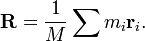# Center of mass of two extended bodies

aftershock

## Homework Statement

Consider a system comprising two extended bodies, which have masses M1 and M2 and centers of mass at R1 and R2. Prove that the CM of the whole system is at

[M1R1+M2R2] / [M1+M2 ]

## Homework Equations

Definition of CM## The Attempt at a Solution

First I looked at the numerator of the equation given in the problem. If I replace R1 with the definition then M1 cancels. The same logic applies to the second term in the numerator. This leaves me with just the summation of all the mr terms for the particles comprising mass 1 and 2 then in the denominator I have the total mass of the system. So the equation given is now in the form of the definition? I feel like this is trivial, but the book has it marked as a harder problem. I feel like I'm missing something and it can't be done this easily. Is my method correct?